Next: Remark Up: What mcfgen does for Previous: Initial processing for each   Contents

## Loop over the reactions

We have mentioned that for each target the routine trgdat transfers the corresponding data from the library' file to the scratch file endata'. The code mcfgen reads data from the file endata', processes it, and prints the results to the output file mcf.asc'. For a given reaction the order of the processing is according to the data type as given by the identifier I_number. For the different values of I_number the various types of data are as follows. Note that no single reaction has all of this data.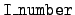= 0:
The original data in the library' file is an array of pairs (incident energy, reaction cross section). The subroutine iequ0 computes and prints flux-weighted averages over the groups g,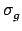=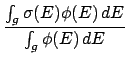.

The integration is done by the trapezoid rule. The flux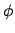is usually constant over each group. The code also calculates and prints out the total available energy.= 1:
The data consists of pairs (energy deposited to secondary particle, probability density) for a sequence of incident energies and cosines of the collision angle. Depending on the kinematics type, this data may be in the laboratory or the center-of-mass system. The subroutine iequ1 computes boundaries for equiprobable cosine bins, and it prints them. See Section 4.2.= 3:
The data consists of pairs (energy of the secondary particle, probability density) for a sequence of incident energies and cosines of the collision angle. This data is in the center-of-mass system. The subroutine iequ3 computes boundaries for joint equiprobable cosine bins and energy bins, and it prints them. This type of data is given only for the 9Be (n, 2n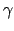)2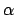reaction and for the Production Cross Section Library. Note that this block of data is not self-sufficient--the angular-distribution data (= 1) must be present and must be consistent. The= 1 and 3 data together provide joint (energy, angle) probability distributions.= 4:
The data consists of pairs (energy of secondary, probability density) for a sequence of incident energies. This data is in the laboratory system. The subroutine iequ4 computes equiprobable energy bins and writes them (along with the incident energies) to the mcf.asc' output file.= 7 or 9:
The cases of data type 7 and 9 are treated together by the subroutine iequ7or9. In both cases the data is an array of pairs (incident energy, multiplicity). For data type 7 the particles are fission neutrons, and for type 9 the data gives the multiplicity of emitted photons. The fission neutrons may be prompt or delayed. The code computes average multiplicities over the energy groups g, weighted by the flux and reaction cross section,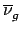=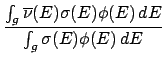,

It then prints the results to the mcf.asc' file.= 10:
The data in the library' file consists of pairs (energy, energy deposition to secondary particle). This data may be in the center-of-mass system or the laboratory system, depending on the kinematics type of the reaction. The subroutine iequ10 computes the average energy deposited in each energy group g,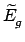=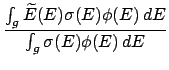,

and it prints the results to the mcf.asc' file.= 12:
This flag is used only for making Production Cross Section files. When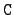= 5 the library' file contains pairs (E, Q), where E is the incident energy and Q the energy available from non-elastic reactions. The code computes and prints the group averages

Qg =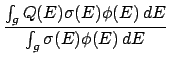.

This processing is done inside the subroutine iequ0.Next: Remark Up: What mcfgen does for Previous: Initial processing for each   Contents